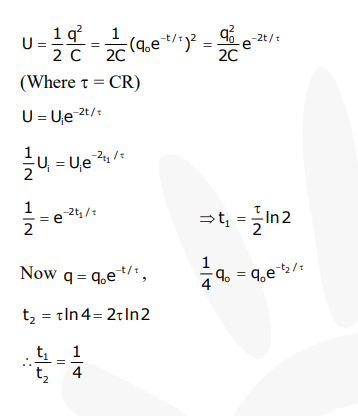Deepak Scored 45->99%ile with Bounce Back Crack Course. You can do it too!

# Let C be the capacitance of a capacitor discharging through a resistor R.

Question:

Let $\mathrm{C}$ be the capacitance of a capacitor discharging through a resistor $R$. Suppose $\mathrm{t}_{1}$ is the time taken for the energy stored in the capacitor to reduce to half its initial value and $t_{2}$ is the time taken for the charge to reduce to one-fourth its initial value. Then the ratio $t_{1} / t_{2}$ will be :

1. 1

2. 1/2

3. 1/4

Correct Option: , 3

Solution: